## A stoppered test tube traps 25.0 cm3 of air at a pressure of 1.00 atm and temperature of 18OC. The cylindrically shaped stopper at the test

Question

A stoppered test tube traps 25.0 cm3 of air at a pressure of 1.00 atm and temperature of 18OC. The cylindrically shaped stopper at the test tube’s mouth has a diameter of 1.20 cm and will “pop off” the test tube if a net upward force of 11.5 N is applied to it.
To what temperature would one have to heat the trapped air in order to “pop off” the stopper? Assume the air surrounding the test tube is always at a pressure of 1.00 atm.

in progress 0
2 months 2021-07-24T09:28:49+00:00 1 Answers 1 views 0

1. Explanation:

The pressure of an object is calculated as follows: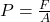The area of test tube stopper is A= π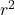Since radius is half the diameter we have the following:

R  =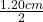=  0.60 cm

Thus the area of the test tube becomes:

A = π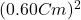= π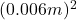= 1.14 *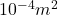Now the force from the outside of the tube.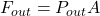=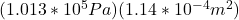= 11.55N

The net upward force on the stopper is equal to the difference of force exerted by pressure from inside of the tube and that from the outside.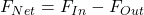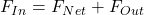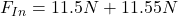= 23.1 N